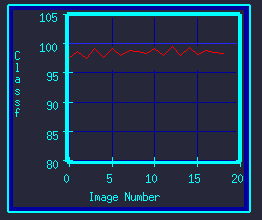The XY-Graph gadget displays vector data as an XY-Graph. It consists of four routines: plot_init - Sets up initial display background, axes, and title labels. plot_axis_x - Puts numbers on the X-axis (horizontal-axis). plot_axis_y - Puts numbers on the Y-axis (vertical-axis). plot_update - Draw curves/lines in the plot-area.```
may exceed the display range without worry of drawing outside the plotting
area.

The formal definitions are:

plot_init( char *obj_name, char *xtitle, char *ytitle )

The obj_name is the name of the box where the graph should be
displayed.  This is normally, MY_NAME.  The xtitle and ytitle
are the axes labels that should displayed on the graph for the
two axes respectively.  For example, "Time", vs "Temperature".
The plot_init routine is usually called once at startup.

It could be called again if the user resizes the simulation window,
by detecting a changed value to the global integer, redraw.

The graph is automatically scaled to fit over the box that is
pointed to by the obj_name/MY_NAME parameter.

plot_axis_y( char *obj_name, float ymin, float ymax, int nogrid,
int scolor, int gcolor )

The obj_name is the name of the box where the graph should be
displayed.  This is normally, MY_NAME.  The ymin and ymax parameters
are the ranges of the y-axis.  The nogrid flag controls whether
grid lines are drawn.  0 turns grids off, while 1 activates it.
The color of the axis numbers is set by scolor.  The color of the
grid lines is set by gcolor.

This routine can be called if the y-axis range changes
during the simulation.  It should be called first with the
old ranges and black colors to erase the old numbers, before
calling it with the new ranges.

plot_axis_x( char *obj_name, float xmin, float xmax, int nogrid,
int scolor, int gcolor )

Like plot_axis_y, but for X-axis (horizontal axis) numbering
and grid lines.

Often, one axis stays fixed, such as the Y-axis, while the
other moves, such as the X-axis.  The axes can be updated
individually, as needed, by these routines.

plot_update( char *obj_name, float xmin, float xmax, float ymin,
float ymax, struct plot_record *plot_data, int color )

The obj_name is the name of the box where the graph should be
displayed.  This is normally, MY_NAME.  The ymin, ymax, xmin,
and xmax parameters are the ranges of the y- and x-axes
respectively.  The plot_data is a pointer to a linked-list
containing the data points to be plotted.  It should have the
following structure:

struct plot_record
{
float x, y;
struct plot_record *nxt;
};

The color parameter controls the color of the line drawn.

Multiple plot lines can be draw with this routine by calling
with each data-set separately.  They can each be drawn with
different colors.

If the axis is moved (range is changed), plot_update should be
called with the old data and range but with black color to
erase the old curve.  Then re-called with the new data, ranges,
and non-black color to draw the new curve.

Example:

/* Display initial graph panel with borders and titles. */
plot_init( MY_NAME, "X-Axis", "Y-Values" );
plot_axis_x( MY_NAME, 0.0, 105.0, 0, Gold, Blue );
plot_axis_y( MY_NAME, 50.0, 250.0, 0, Gold, Blue );

/* Convert array of data, of length N, into plot_record. */
grph = 0;  /* Initialize head of list to zero. */
for (i=0; i!=N; i++)
{
tmpgrph = (struct plot_record *)malloc(sizeof(struct plot_record));
tmpgrph->nxt = grph;   /* Push new element onto list by attaching */
grph = tmpgrph;        /* list to element, and replacing list's head. */
grph->x = (float)i;    /* Set new element's data values. */
grph->y = vector[i];
}

/* Display the data. */
plot_update( MY_NAME, 0.0, N, 50.0, 250.0, grph, Green );

/* Free the old data. */
while (grph!=0) { tmpgrph = grph->nxt; free(grph); grph = tmpgrph; }

Convenient general-purpose oscilloscope-style box-models are part
of the Vector-library.  Device-type, XYgraph, accepts vector-data on
its input port and displays the data updated on graphs.  The XYgraph2
model is like XYgraph, but accepts and displays two vectors.
These models use the above routines, and serve as more complete
examples of how to use them.  They are also useful self-contained
display-boxes for use with the vector elements without need for
manipulating the basic xy-plot gadget routines.

```

### Refreshing Graph Background After Redraw Events

Whenever the screen is redrawn for any reason, you will lose the XY-graph background that you originally initiallized. You can detect such redraw events and restore your graph's background and axes labels by the following: Detecting ReDraw Events.